Home

# On the ℱϕ-Hypercentre of Finite Groups

## Abstract

Let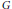$G$ be a finite group and let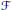$\mathcal{F}$ be a class of groups. Then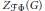${{Z}_{\mathcal{F}\Phi }}\left( G \right)$ is the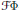$\mathcal{F}\Phi$ -hypercentre of$G$ , which is the product of all normal subgroups of$G$ whose non-Frattini$G$ -chief factors are$\mathcal{F}$ -central in$G$ . A subgroup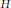$H$ is called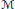$\mathcal{M}$ -supplemented in a finite group$G$ if there exists a subgroup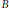$B$ of$G$ such that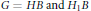$G\,=\,HB\,\text{and}\,{{H}_{1}}B$ is a proper subgroup of$G$ for any maximal subgroup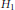${{H}_{1}}$ of$H$ . The main purpose of this paper is to prove the following: Let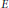$E$ be a normal subgroup of a group$G$ . Suppose that every noncyclic Sylow subgroup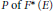$P\,\text{of}\,{{F}^{*}}\left( E \right)$ has a subgroup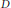$D$ such that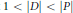$1\,<\,\left| D \right|\,<\left| P \right|$ and every subgroup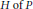$H\,\text{of}\,P$ with order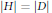$\left| H \right|\,=\,\left| D \right|$ is$\mathcal{M}$ -supplemented in$G$ , then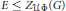$E\,\le \,{{Z}_{\mathcal{U}\Phi }}\left( G \right)$ .

• # Send article to Kindle

Note you can select to send to either the @free.kindle.com or @kindle.com variations. ‘@free.kindle.com’ emails are free but can only be sent to your device when it is connected to wi-fi. ‘@kindle.com’ emails can be delivered even when you are not connected to wi-fi, but note that service fees apply.

Find out more about the Kindle Personal Document Service.

On the ℱϕ-Hypercentre of Finite Groups
Available formats
×

# Send article to Dropbox

To send this article to your Dropbox account, please select one or more formats and confirm that you agree to abide by our usage policies. If this is the first time you use this feature, you will be asked to authorise Cambridge Core to connect with your <service> account. Find out more about sending content to Dropbox.

On the ℱϕ-Hypercentre of Finite Groups
Available formats
×

# Send article to Google Drive

To send this article to your Google Drive account, please select one or more formats and confirm that you agree to abide by our usage policies. If this is the first time you use this feature, you will be asked to authorise Cambridge Core to connect with your <service> account. Find out more about sending content to Google Drive.

On the ℱϕ-Hypercentre of Finite Groups
Available formats
×

## Corresponding author

L. Miao is the corresponding author

## Footnotes

Hide All

This research is supported by NSFC (Grant #11271016), the Postgraduate Innovation Project of Jiangsu Province (No. CXZZ13–0890), and the Natural Science Fund for Colleges and Universities in Anhui Province (Grant #KJ2013B138).

## References

Hide All
MathJax
MathJax is a JavaScript display engine for mathematics. For more information see http://www.mathjax.org.

# On the ℱϕ-Hypercentre of Finite Groups

## Metrics

### Full text viewsFull text views reflects the number of PDF downloads, PDFs sent to Google Drive, Dropbox and Kindle and HTML full text views.

Total number of HTML views: 0
Total number of PDF views: 0 *Loading metrics...

### Abstract viewsAbstract views reflect the number of visits to the article landing page.

Total abstract views: 0 *Loading metrics...

* Views captured on Cambridge Core between <date>. This data will be updated every 24 hours.

Usage data cannot currently be displayed